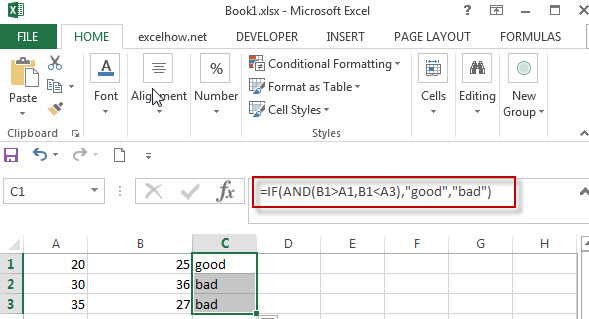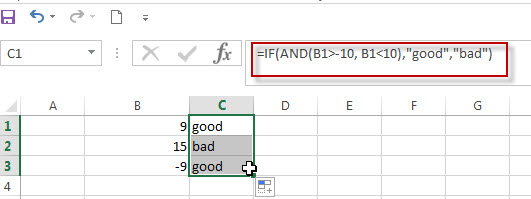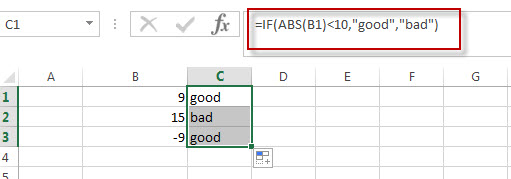# Excel IF Function With Numbers

Normally, If you want to write an IF formula for numbers in combining with the  logical operators in excel, such as: greater than, less than, greater than or equal to, less than or equal to, equal to, not equal to.etc.

The generic IF formula with logical operator for number values is as below:

`=IF(B1>10,”good”,”bad”)`

## Excel IF formula for range of numbers

If you want to check if a cell values is between two values or checking for the range of numbers or multiple values in cells, at this time, we need to use AND or OR logical function in combination with the logical operator and IF function.

For example, you need to check if the value in cell B1 is between values in Cell A1 and A3, then we can write down the following excel IF formula:

`=IF(AND(B1>A1,B1<A3),"good","bad")`

We can enter the above formula into the formula bar in the cell C1 and then press the Enter key. And rag the Fill Handle to the range C1:C3.it will apply for the other cells for this formula.## Excel IF formula for negative numbers

If you want to create an IF formula to display some text string in cells if the value in cell falls between the range negative numbers -10 to positive numbers 10.

Actually, whatever negative numbers or positive numbers, we still can use AND or OR logical function to create a logical test in IF formula. we can write down the following IF formula:

`=IF(AND(B1>-10, B1<10),”good”,”bad”)`Also, we can use another excel function ABS() to achieve the same results. Let’s see the below IF formula in combination with ABS function.

`=IF(ABS(B1)<10,”good”,”bad”)`### Related Formulas

• Excel IF formula with operator : greater than,less than
Now we can use the above logical test with greater than operator. Let’s see the below generic if formula with greater than operator:=IF(A1>10,”excelhow.net”,”google”) …
• Excel IF function with text values
If you want to write an IF formula for text values in combining with the below two logical operators in excel, such as: “equal to” or “not equal to”.
• Excel IF function with Dates
If you have a list of dates and then want to compare to these dates with a specified date to check if those dates is greater than or less than that specified date. …
• Excel IF Function With Numbers
If you want to check if a cell values is between two values or checking for the range of numbers or multiple values in cells, at this time, we need to use AND or OR logical function in combination with the logical operator and IF function…

### Related Functions

• Excel AND function
The Excel AND function returns TRUE if all of arguments are TRUE, and it returns FALSE if any of arguments are FALSE.The syntax of the AND function is as below:= AND (condition1,[condition2],…) …
• Excel IF function
The Excel IF function perform a logical test to return one value if the condition is TRUE and return another value if the condition is FALSE….
• Excel nested if function
The nested IF function is formed by multiple if statements within one Excel if function. This excel nested if statement makes it possible for a single formula to take multiple actions…
• Excel ABS function
The Excel ABS function returns the absolute value of a number